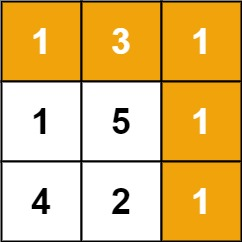64. Minimum Path Sum

Given a `m x n` `grid` filled with non-negative numbers, find a path from top left to bottom right, which minimizes the sum of all numbers along its path.

Note: You can only move either down or right at any point in time.

Example 1:```Input: grid = [[1,3,1],[1,5,1],[4,2,1]]
Output: 7
Explanation: Because the path 1 → 3 → 1 → 1 → 1 minimizes the sum.
```

Example 2:

```Input: grid = [[1,2,3],[4,5,6]]
Output: 12
```

Constraints:

• `m == grid.length`
• `n == grid[i].length`
• `1 <= m, n <= 200`
• `0 <= grid[i][j] <= 100`

64. Minimum Path Sum
``````struct Solution;

impl Solution {
fn min_path_sum(grid: Vec<Vec<i32>>) -> i32 {
let n = grid.len();
let m = grid.len();
let mut dp: Vec<Vec<i32>> = vec![vec![0; m]; n];
dp = grid;
for j in 1..m {
dp[j] = dp[j - 1] + grid[j];
}
for i in 1..n {
dp[i] = dp[i - 1] + grid[i];
}
for i in 1..n {
for j in 1..m {
dp[i][j] = i32::min(dp[i - 1][j], dp[i][j - 1]) + grid[i][j];
}
}
dp[n - 1][m - 1]
}
}

#[test]
fn test() {
let grid: Vec<Vec<i32>> = vec_vec_i32![[1, 3, 1], [1, 5, 1], [4, 2, 1]];
let res = 7;
assert_eq!(Solution::min_path_sum(grid), res);
}
``````